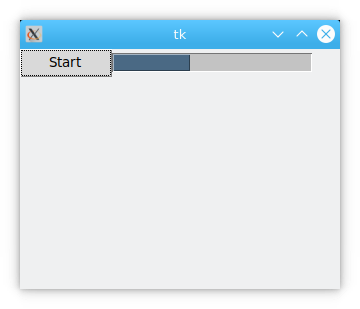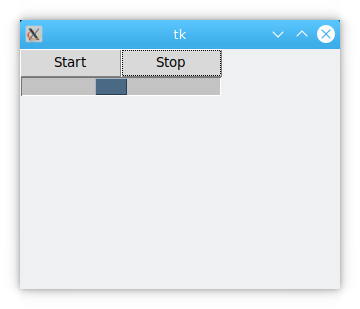# tkinter progressbar

This article shows the tkinter progressbar. The purpose of the progress bar is to prompt the user for progress information. This can be done in two ways.
For example, the progress of the program being installed, typically from 0% to 100%.

## tkinter progressbar

The program below creates a progressbar in a tkinter window. If you click the button you’ll see the progressbar slowly increase to 100.

``````import tkinter as tk
from tkinter import ttk
import time
def increment(*args):
for i in range(100):
p1["value"] = i+1
root.update()
time.sleep(0.1)
root = tk.Tk()
root.geometry('320x240')
p1 = ttk.Progressbar(root, length=200, cursor='spider',
mode="determinate",
orient=tk.HORIZONTAL)
p1.grid(row=1,column=1)
btn = ttk.Button(root,text="Start",command=increment)
btn.grid(row=1,column=0)
root.mainloop()``````

Results.### length

Sets the length of the progress bar. The default unit is pixels, but it can also be other units. See section 3.3.1 for detailed unit descriptions.

``````import tkinter as tk
from tkinter import ttk
import time
def increment(*args):
for i in range(100):
p1["value"] = i+1
root.update()
time.sleep(0.1)
root = tk.Tk()
root.geometry('360x240')
p1 = ttk.Progressbar(root, length='2i', mode="determinate",
orient=tk.HORIZONTAL)
p1.grid(row=1,column=1)
btn = ttk.Button(root,text="Start",command=increment)
btn.grid(row=1,column=0)
root.mainloop()``````

Note: The length is ‘2i’, which means it is 2 inches long.

### max

Defines the maximum scale of the progress bar. The default value is 100.

``````import tkinter as tk
from tkinter import ttk
import time
def increment(*args):
for i in range(100):
p1["value"] = i+1
root.update()
time.sleep(0.1)
root = tk.Tk()
root.geometry('320x240')
p1 = ttk.Progressbar(root, length=200, mode ="determinate",
maximum=200,orientation=tk.HORIZONTAL)
p1.grid(row=1,column=1)

btn = ttk.Button(root,text="Start",command=increment)
btn.grid(row=1,column=0)
root.mainloop()``````

### mode

Set the mode of the progress bar. There are two modes for the progress bar: ‘determinate’ and ‘indeterminate’

(1) determinate

For the ‘determinate’ mode, under the program’s control, the progress bar instructions will start at 0 and move to the Maximum scale, then stop. This is used to know the exact progress data. For example, if you read a file, you know the size of the file, and you can use the percentages to show the degree of completion.

(2) indeterminate

For ‘indeterminate’, the scale of the progress bar is reciprocating, indicating that a process is in progress . This situation is used when the exact progress data is not known, i.e., the status of completion is not known. For example, if a query to the database returns a large number of results and the program has no way of knowing in advance how many results will be returned.

``````import tkinter as tk
from tkinter import ttk
import time
def increment(*args):
p1.start()
root = tk.Tk()
root.geometry('320x240')
p1 = ttk.Progressbar(root, length=200, mode ="indeterminate",
maximum=200,orientation=tk.HORIZONTAL)
p1.grid(row=1,column=1)
btn = ttk.Button(root,text="Start",command=increment)
btn.grid(row=1,column=0)
root.mainloop()    ``````

Description: indeterminate is called by using the start() method of the progressbar control.

### orient

Set the direction of the progress bar in two formats: HORIZONTAL and VERTICAL.

### style

Set the appearance of the progress bar. See Section 23.2 for specific usage.

### takefocus

Sets whether or not the progress bar gets input focus.

19.1.8 variable

A variable related to the progress bar, IntVar(), which can be used to set or get the current value of the progress bar.

### value

The current value of the progress bar.

### Functions

Function Description

• start(interval=None) Automatically adjusts the position of the progress bar. Adjusts the position of the progress bar by starting a cyclic timer event with a defined step size. The interval of the timer is set by the interval parameter. The interval is set in milliseconds. The default interval is 50 milliseconds.

• step(amount=None) Adjusts the step length of the progress bar each time, default is 1.0.

• stop() Stops the timer, stops the auto-adjustment of the progress bar.

#### start(interval=None)

Auto-adjustment of the progress bar starts at a default interval of 50 milliseconds. Auto-adjustment is achieved by starting a loopable timer. Each step of adjustment is implemented by the step() function.

#### step(amount=None)

Defines the step size for each progress bar adjustment. The default is 1.0, but other values can be set by setting amount.

#### stop

Stops the progress bar from being adjusted.

``````import tkinter as tk
from tkinter import ttk
import time

def start(*args):
p1.start(30)
p1.start(30)
def stop(*args):
value=p1['value']
p1.stop()
p1['value']=value
root = tk.Tk()
root.geometry('320x240')
p1 = ttk.Progressbar(root, length=200,
mode="indeterminate",
orient=tk.HORIZONTAL)
p1.grid(row=1,column=0,columnspan=2)

btn_start = ttk.Button(root,text="Start",command=start,width=10)
btn_stop = ttk.Button(root,text="Stop",command=stop,width=10)

btn_start.grid(row=0,column=0)
btn_stop.grid(row=0,column=1)

root.mainloop()``````### Properties

The properties of the progressbar are:

Property Description
cursor The shape of the progress bar when the mouse is inside it.
Length The length of the progress bar.
maximum The maximum scale value of the progress bar.
mode The mode of the progress bar. There are two modes: ‘determinate’ and ‘indeterminate’.
orient The direction of the progress bar, either HORIZONTAL or VERTICAL.
style Defines the appearance of the progress bar.
variable The variable associated with the progress bar. You can set or get the current value of the progress bar
value Set or get the current value of the progress bar.